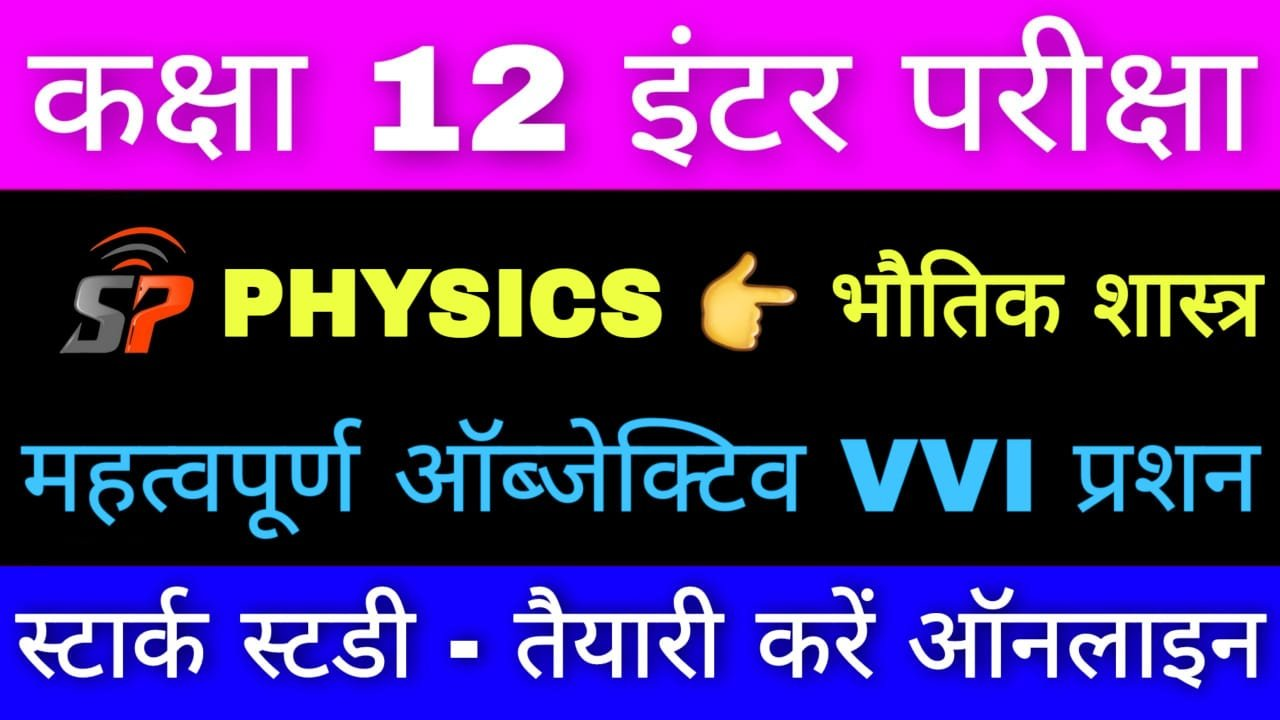Class 12th Physics Chapter 10 Wave Optics VVI Objective Question

Wave Optics

1. Electromagnetic wave can be Polarised by

(A) a lens

(B) a mirror

(C) a polaroid

(D) a prism

2. Bonding of light rays at sharp obstacles is called :

(A) Refraction

(B) Diffraction

(C) Interference

(D) Polarisation

3. If the ratio of amplitudes of two interfering waves is 4:3, then the ratio of maximum and minimum intensity is :

(A) 16:9

(B) 9:16

(C) 49:1

(D) 1:49

4. Which of the following could not be explained by wave nature of light?

(A) Reflection de

(B) Refraction

(C) Diffraction

(D) Photo-electric effect

5. The phenomenon which exhibits that a certain wave is transverse is :

(A) Dispersion

(B) Diffraction

(C) Interference

(D) Polarization

6. A rainbow is a natural example of

(A) refraction of light

(B) reflection of light

(C) refraction, reflection and dispersion of light

(D) None of these

7. Electromagnetic waves can be polarized by

(A) Lens

(B) mirror

(C) polaroid

(D) prism

8. When a ray of light enters a glass slab its wavelength

(A) decreases

(B) increases

(C) remains unchanged

(D) data is not sufficient

9. Cause of mirage is

(A) refraction and total internal reflection

(B) diffraction

(C) scattering

(D) interference

10. The reason of blue colour of the sky is

(A) scattering

(B) interference

(C) Polarization

(D) diffraction

11. The dark lines in solar spectrum are known as

(A) Fraunofer lines

(B) Telluric lines

(C) Both (A) and (B)

(D) None of these

12. Pure spectrum can be obtained using

(A) microscope

(B) spherometer

(C) spectrometer

(D) prism only

13. In the spectrum of white light obtained by a prism, the colour showing minimum deviation is

(A) yellow

(B) red

(C) blue

(D) violet

14. In Fraunhofer class of diffraction, the source of light is placed

(A) at finite distance from the obstacle

(B) in close contact with the source

(C) at infinite distance form the source

(D) None of these

15. In a plane polarised light, the vector of electric field intensity vibrate in

(A) all directions

(B) a single plane

(C) mutually perpendicular directions

(D) None of these

16. A soap bubble appears colourful in white light because of

(A) polarization of light

(B) scattering of light

(C) refraction of light

(D) interference of light

17. Two virtual and coherent sources are produced in

(A) Young’s double-slit experiment

(B) Lyod mirror

(C) Fresnel’s biprism

(D) all of these

18. Huygens secondary wave principle is used to

(A) new geometrical position of the wavefront

(B) explain the superposition principle of waves

(C) explain the interference of light

(D) explain the polarization of light

19. Light consists of vibrations of

(A) Ether particles

(B) air particles

(C) electric and magnetic fields

(D) None of these

20. According to wave motion-theory of light, the colour of light is decided by

(A) amplitude

(B) speed of wave

(C) frequency

(D) wavelength

21. The concept of secondary wavelets was given by

(A) Fresnel

(B) Maxwell

(C) Huygens

(D) Newton

22. When a polaroid is rotated about the incident beam, the intensity of light passed does not change. It so happens when the incident beam of light is

(A) fully plane polarized

(B) partly plane polarized

(C) unpolarized

(D) None of these

23. Two sources of waves are said to be coherent of

(A) their amplitudes are equal

(B) they produce waves of equal wavelengths

(C) they produce waves of equal velocities

(D) they have constant phase difference

24. The tangent of polarization angle is equal to the refractive index. This law is known as

(A) Malus law

(B) Brewster’s law

(C) Bragg’s law

(D) Compton’s law

25. If the wavelength of light in Young’s experiment is doubled, the fringe width

(A) remains the same

(B) gets doubled to

(C) is halved

26. The colour of a thin film is due to

(A) scattering

(B) interference

(C) dispersion

(D) diffraction

27. Optical fibre communication is based on which of the following phenomena :

(A) Total Internal reflection

(B) Scattering

(C) Reflection

(D) Interference